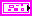Background Image Property

FlexRIO Help

Edition Date: November 2015

Part Number: 372614J-01

»View Product Info

Short Name: BackgroundImage

Requires: Base Development System

Class: Pane Properties

To Use: Create a property.

Sets the background image the pane uses. LabVIEW supports BMP, JPEG, and PNG graphic formats for background images.

This property is similar to the Browse option on the Appearance page of the Pane Properties dialog box.

If you want to use a picture to set the image, use the Picture to Pixmap VI to convert the data to an image data cluster before using this method. To use a file to set the image, use the Read JPEG File, Read PNG File, or Read BMP File VIs to convert the data to an image data cluster before using this method.

Elements

Name Description
Image Type Reserved for future use.
Image Depth Specifies the color depth of the image, which is the number of bits to use to describe the color of each pixel in the image. Valid values include 1, 4, 8, and 24 bits per pixel. Image Depth affects how LabVIEW interprets the values of Image and Colors.
Image A 1-D array of bytes that describes the color of each pixel in the image in raster order. The value of Image Depth determines how LabVIEW interprets the value of this input.

If Image Depth is 24, each pixel has three bytes to describe its color. The first byte for each pixel describes the red value, the second byte describes the green value, and the third byte describes the blue value.

If Image Depth is 8, each pixel has one byte to describe its color. The value of each bit corresponds to an element in Colors, which stores 32-bit RGB values where the most-significant byte is zero, followed in order by red, green, and blue values. Valid values include 0 through 255.

If Image Depth is 4, the behavior is similar to when Image Depth is 8 except valid values in image include 0 through 15.

If Image Depth is 1, any value of zero in Image corresponds to element 0 in Colors. All other values correspond to element 1 in Colors.
Mask An array of bytes in which each bit describes mask information for a pixel. The first byte describes the first eight pixels, the second byte describes the next eight pixels, and so on. If a bit is zero, LabVIEW draws the corresponding pixel as transparent. If the array is empty, LabVIEW draws all pixels without transparency. If the array does not contain a bit for each pixel in the image, LabVIEW draws any pixels missing from the array without transparency.
Colors An array of RGB color values that correspond to the values in image. The value of Image Depth determines how LabVIEW interprets the value of this input.

If Image Depth is 24, LabVIEW ignores this input.

If Image Depth is 8, the array can have 256 elements.

If Image Depth is 4, the array can have 16 elements.

If Image Depth is 1, the array can have 2 elements.
Rectangle A cluster that contains coordinates that describe the bounding rectangle in which you want to draw the image. The VI clips the image to the width and height of the rectangle. The bottom and right edges of the rectangle bounds do not contain image pixels. Horizontal coordinates increase to the right, and vertical coordinates increase to the bottom.
• Left—The horizontal coordinate of the left edge of the rectangle.
• Top—The vertical coordinate of the top edge of the rectangle.
• Right—The horizontal coordinate of the right edge of the rectangle.
• Bottom—The vertical coordinate of the bottom edge of the rectangle.

Remarks

The following table lists the characteristics of this property.

 Data typePermissions Read/Write Available in Run-Time Engine Yes Available in Real-Time Operating System Yes Settable when the VI is running Yes Loads the front panel into memory Yes Need to authenticate before use No Loads the block diagram into memory No Remote access allowed Yes